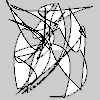Create a collection of quadratic vertices.

## Examples#import numpy as np

def setup():
py5.size(100, 100, py5.P2D)
random_quadratic_vertices = 100 * np.random.rand(25, 4)
s = py5.create_shape()
with s.begin_shape():
s.vertex(py5.width / 2, py5.height / 2)
py5.shape(s)


## Description#

Create a collection of quadratic vertices. The purpose of this method is to provide an alternative to repeatedly calling Py5Shape.quadratic_vertex() in a loop. For a large number of quadratic vertices, the performance of quadratic_vertices() will be much faster.

The coordinates parameter should be a numpy array with one row for each quadratic vertex. The first few columns are for the control point and the next few columns are for the anchor point. There should be four or six columns for 2D or 3D points, respectively.

Drawing 2D bezier curves requires using the P2D renderer and drawing 3D bezier curves requires using the P3D renderer. When drawing directly with Py5Shape objects, bezier curves do not work at all using the default renderer.

## Signatures#

quadratic_vertices(
coordinates: npt.NDArray[np.floating],  # 2D array of quadratic vertex coordinates with 4 or 6 columns for 2D or 3D points, respectively
/,
) -> None


Updated on June 26, 2023 01:48:07am UTC